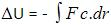Courses

# Test: Conservative Forces

## 10 Questions MCQ Test Physics For JEE | Test: Conservative Forces

Description
This mock test of Test: Conservative Forces for JEE helps you for every JEE entrance exam. This contains 10 Multiple Choice Questions for JEE Test: Conservative Forces (mcq) to study with solutions a complete question bank. The solved questions answers in this Test: Conservative Forces quiz give you a good mix of easy questions and tough questions. JEE students definitely take this Test: Conservative Forces exercise for a better result in the exam. You can find other Test: Conservative Forces extra questions, long questions & short questions for JEE on EduRev as well by searching above.
QUESTION: 1

### Work done by gravitational force on a man, in lifting a bucket out of the well by rope tied to the bucket is

Solution:

When the man pulls the bucket outside the well the gravitational potential of the man + bucket system increases and hence the work done by the gravitational force is negative.

QUESTION: 2

### For a conservative force, F is equal to

Solution:

Potential energy at a point is defined in terms the amount of work done, which is defined in terms of force and thus we get potential as an integral of force applied over some position x. Hence F(x) = -dU(x) / dx which is the differentiation of potential energy wrt position.

QUESTION: 3

### Work done in motion of the body over a closed loop for conservative forces is

Solution:
QUESTION: 4

The conservative frictional force always acts ____________ to the surface of the application of the friction.

Solution:

The friction is the phenomena that defines that there is a resistance which is present there between the two surfaces. This friction is applied tangentially to the surfaces in contact. Thus the main thing is that the forces on both of the surfaces act tangential to each other.

QUESTION: 5

Work done by or against a conservative force in a round trip is

Solution:

A conservative force is a force with the property that the total work done in moving a particle between two points is independent of the taken path. Equivalently, if a particle travels in a closed loop, the total work done (the sum of the force acting along the path multiplied by the displacement) by a conservative force is zero.

QUESTION: 6

Select the odd one out

Solution:

Frictional force, Air-resistance, viscous force all these forces work against each other i.e., they are opposing forces, while electrostatic force is not a opposing force.

QUESTION: 7

A force which does not depend on the path taken to increase the potential energy is

Solution:

A force is said to be conservative if the work done by or against the force on a body is independent of path followed by the body and depends only on initial and final positions.
Work done by or against the conservative force in moving a particle along a closed path is zero.
Hence C is correct.

QUESTION: 8

Which of the following is not conserved in inelastic collision?

Solution:

In an inelastic collision, the force of reformation is not equal to the force of deformation and thus some amount of energy is lost. But still as no external force acts upon the system momentum is still conserved.

QUESTION: 9

When conservative force does positive work on a body, the potential energy of the body:

Solution:

We have a relation between potential energy and work done:The negative sign just shows the inverse relation between potential energy and work done by conservative force.

QUESTION: 10

Which of the following statement is not related to conservative force?

Solution:

A force is said to be conservative if the work done by or against the force on a body is independent of path followed by the body and depends only on initial and final positions.
Work done by or against the conservative force in moving a particle along a closed path is zero.